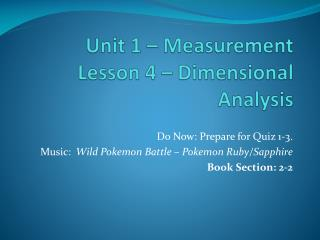DownloadDownload PresentationUnit 1 – Measurement Lesson 4 – Dimensional Analysis

# Unit 1 – Measurement Lesson 4 – Dimensional Analysis

Download Presentation## Unit 1 – Measurement Lesson 4 – Dimensional Analysis

- - - - - - - - - - - - - - - - - - - - - - - - - - - E N D - - - - - - - - - - - - - - - - - - - - - - - - - - -
##### Presentation Transcript

1. Unit 1 – MeasurementLesson 4 – Dimensional Analysis Do Now: Prepare for Quiz 1-3. Music: Wild Pokemon Battle – Pokemon Ruby/Sapphire Book Section: 2-2

2. Dimensional Analysis • Uh… what?

3. Dimensional Analysis • Dimensional analysis is a mathematical technique that allows you to use units to solve problems involving measurements. • Isn’t that what we did last time? We converted units! • Yes, but only with the metric system, and only in one “dimension”… or 1-D. • We converted cm to km, etc. • But how do we convert cm2 to km2 , or cm3 to km3? • Come, let us analyze these dimensions…

4. Dimensional Analysis

5. Problem Solving in Chemistry • The biggest skill that you are going to need in Chemistry is knowing how to convert between units. At least half of the problems we do in this course focus on this skill. • And, unfortunately, it is the skill that causes the most trouble with students. • In science, you treat units just like you would a variable. • Addition • Math: 3x + 2x = 5x • Science: 3 mL + 2 mL = 5 mL • Multiplication • Math: (5x)(2x) = 10x2 • Science: (5 cm)(2 cm) = 10 cm2

6. Conversion Factors • A conversion factor is a fraction using two different units that is equal to 1. • How many hours are in a day? • 24 hours = 1 day • OR • A word of caution – the problems in this section are Algebra I level unit conversion problems, which could be solved by other means. Practicing the chemistry method with these easier problems is what the objective of this lesson is, so that when we do more complicated conversions, you will not be in over your head.

7. Your First Dimensional Analysis Problem • There are 3,600 seconds in an hour. How many seconds are in a day? • Step 1) Write down the given information as a mathematical expression. • Given: 3,600 seconds in an hour. • 3,600 seconds per hour

8. Your First Dimensional Analysis Problem • Step 2) Multiply your given information by a conversion factor that will allow you to cancel unwanted units. • multiplied by or ? • The unwanted unit is hours, which start in the denominator. • So, we must multiply by the conversion factor with hours in the numerator, to cancel hours.

9. Dimensional Analysis Squared and Cubed • Problem 2: How many square centimeters are in a square meter? • Same approach, with a twist. • Given: 1 square meter, or 1 m2. • What is the conversion factor between cm and m? • There are 100 cm in a m • 100 cm = 1 m • Conversion factor: • But, because we are dealing in squares, we need to square the conversion factor. • : The square is applied to everything, including the units!

10. Dimensional Analysis Squared and Cubed • All that’s left is to do the math. • It is a straightforward process that will not steer you wrong once you master it. That is your job now.

11. Why We Square and Cube • Remember that in squares and cubes, we are changing all sides of the square and cube. • Draw a line to represent a foot, and one to represent a yard. • Now, make square feet and square yards. • You should be able to fit 3 squared, or 9 square feet in a square yard.

12. An Example – Why this is important NOW • In November, you will be doing problems that are set up like this: • For your survival in honors chem, you must master this skill. Otherwise, half of this year will be a blur. This is your formal warning.

13. HW 1-4: #12-20 • Week Schedule: • Friday: Quiz 1-4, Density (2-2) • Metric Measurements Lab Due 9/7 • Unit 1 Test (for your agendas) – Thursday, September 9th